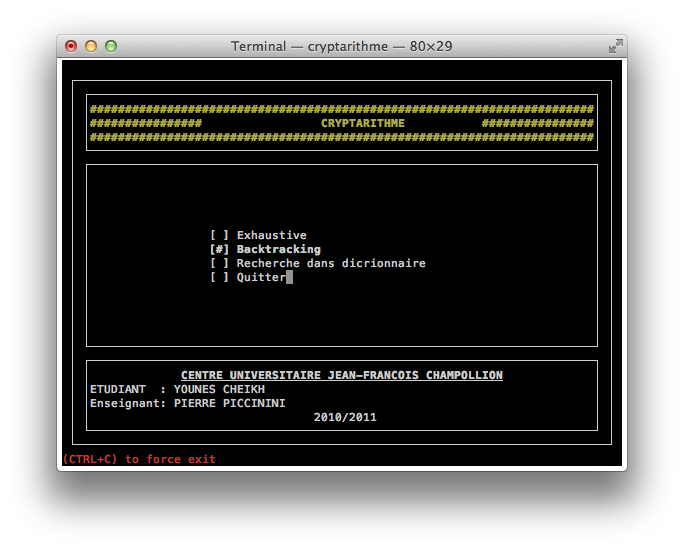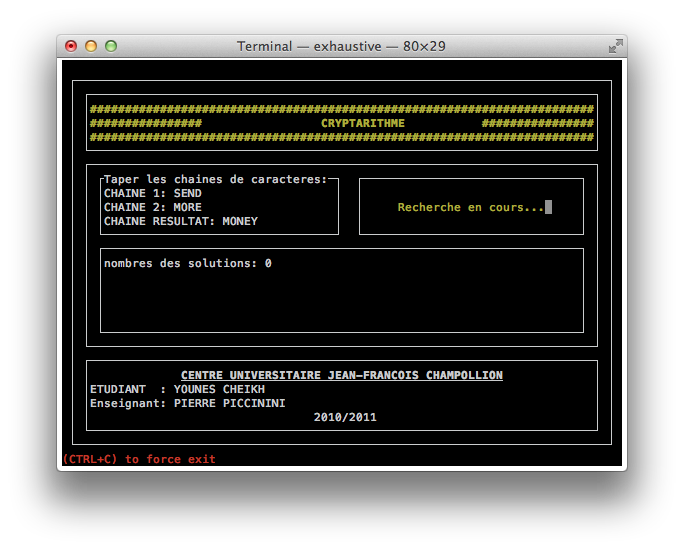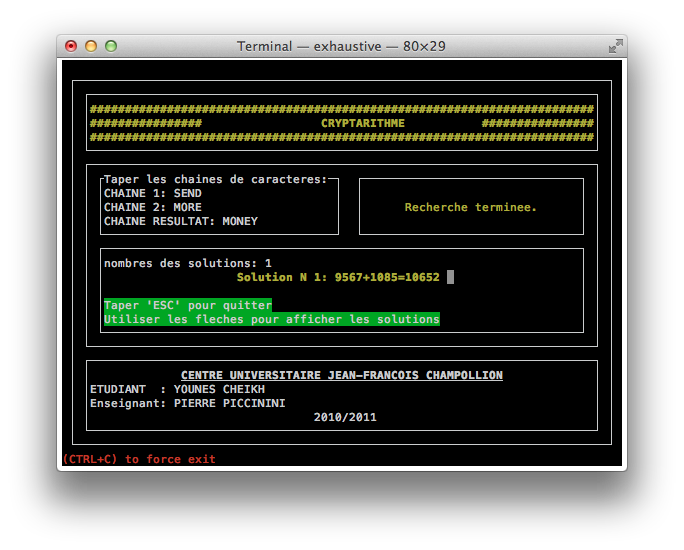Cryptarithms are a type of mathematical puzzle in which the digits are replaced bye symbols (typically letters of the alphabet). For example: 9567 + 1085 = 10652 can be represented like this: abcd + efgb = efcbh The term alphametic is used when the letters from words and phrases.This explains how to use this source code , how to start solving and others… any problems, send an email to the developer .## Compile:

``````make
``````

##Execute:

``````./cryptarithme
``````

choose a methode to solve a cryptarithm, (backtracking or bruteForce) and follow the instructions :)